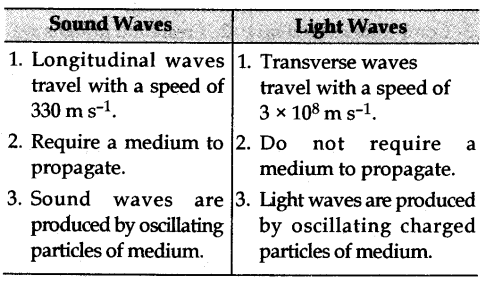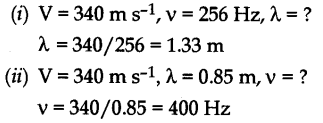# (a) Write two points of difference between sound wave and light wave

(a) Write two points of difference between sound wave and light wave.
(b) Write an expression relating wave velocity, wavelength and frequency if speed of sound in air is 340 m s^-2.
Calculate:
(i) Wavelength when frequency = 256 Hz.
(ii) Frequency when wavelength = 0.85 m.

(a) Two differences are as follows :(b) Velocity of sound = Frequency x Wavelength